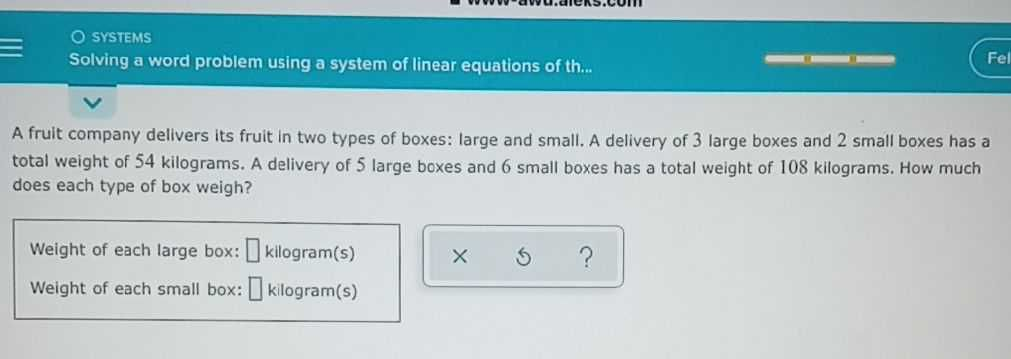### ¿Todavía tienes preguntas de matemáticas?

Pregunte a nuestros tutores expertos
Algebra
PreguntaA frult company delivers its fruit in two types of boxes: large and small. A delivery of $$3$$ large boxes and $$2$$ small boxes has a total weight of $$54$$ kilograms. A delivery of $$5$$ large boxes and $$6$$ small boxes has a total weight of $$108$$ kilograms. How much does each type of box weigh?